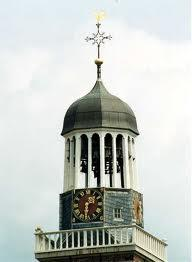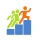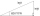# Steeple

Steeple seen from the road at an angle of 75°. When we zoom out to 25 meters, it is seen at an angle of 20°. What is high?

Result

x =  8.5 m

#### Solution:

$x= \dfrac{ 21 \cdot \tan (75^\circ)}{ \dfrac{ \tan (75^\circ)}{\tan (20^\circ)} -1} = 8.5 \ \text{m}$Our examples were largely sent or created by pupils and students themselves. Therefore, we would be pleased if you could send us any errors you found, spelling mistakes, or rephasing the example. Thank you!

Leave us a comment of this math problem and its solution (i.e. if it is still somewhat unclear...):Be the first to comment!Tips to related online calculators

## Next similar math problems:

1. FlowerbedFlowerbed has the shape of an isosceles obtuse triangle. Arm has a size 5.5 meters and an angle opposite to the base size is 94°. What is the distance from the base to opposite vertex?
2. TreeHow tall is the tree that observed in the visual angle of 52°? If I stand 5 m from the tree and eyes are two meters above the ground.
3. MapleMaple peak is visible from a distance 3 m from the trunk from a height of 1.8 m at angle 62°. Determine the height of the maple.
4. Isosceles triangle 10In an isosceles triangle, the equal sides are 2/3 of the length of the base. Determine the measure of the base angles.
5. Theorem proveWe want to prove the sentence: If the natural number n is divisible by six, then n is divisible by three. From what assumption we started?
6. BuildingThe building I focused at an angle 30°. When I moved 5 m building I focused at an angle 45°. What is the height of the building?
7. High wallI have a wall 2m high. I need a 15 degree angle (upward) to second wall 4 meters away. How high must the second wall?
8. ReflectorCircular reflector throws light cone with a vertex angle 49° and is on 33 m height tower. The axis of the light beam has with the axis of the tower angle 30°. What is the maximum length of the illuminated horizontal plane?
9. Right triangleLadder 16 feet reaches up 14 feet on a house wall. The 90-degree angle at the base of the house and wall. What are the other two angles or the length of the leg of the yard?
10. 30-60-90The longer leg of a 30°-60°-90° triangle measures 5. What is the length of the shorter leg?
11. Reference angleFind the reference angle of each angle:
12. Cable car 2Cable car rises at an angle 41° and connects the upper and lower station with an altitude difference of 1175 m. How long is the track of cable car?
13. StepsFind the height between the two floors if you know that the number of steps between the two floors is 18, the gradient is 30º and the length of the step is 28.6 cm. Report the result in centimeters to the nearest centimeter.
14. Right triangleCalculate the length of the remaining two sides and the angles in the rectangular triangle ABC if a = 10 cm, angle alpha = 18°40'.
15. One sideOne side is 36 long with a 15° incline. What is the height at the end of that side?
16. TrigonometryIs true equality? ?
17. TriangleCalculate the area of ​​the triangle ABC if b = c = 17 cm, R = 19 cm (R is the circumradius).